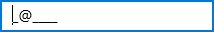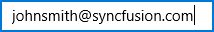10 May 20214 minutes to read

Each `MaskType` has different set of mask elements that are combined to form a mask expression. Based on the complexity and usage, mask types are classified as,

1. Simple
2. Regular
3. RegEx

## Simple

Expressions that are generated with very simple mask elements come under this group. This is mainly used for fixed length inputs. For example: Phone number.

Elements Description
0 Digit, required. This element accepts any single digit between 0 and 9.
9 Digit or space, optional.
# Digit or space, optional. Plus (+) and minus (-) signs are allowed.
L Letter, required. Restricts input to the ASCII letters a-z and A-Z. This mask element is equivalent to [a-z A-Z] in regular expressions.
? Letter, optional. Restricts input to the ASCII letters a-z and A-Z. This mask element is equivalent to [a-z A-Z]? in regular expressions.
C Character, optional.
A Alphanumeric, required.
< Shift down. Converts all the characters that follow to lowercase.
> Shift up. Converts all the characters that follow to uppercase.

### Example

``<Input:SfMaskedEdit MaskType="Simple" Mask="00/00/0000" Width="255" Height="46"/>``
``````SfMaskedEdit maskededit = new SfMaskedEdit();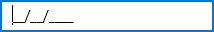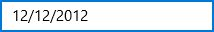## Regular

Regular mask supports simple regular expression with below listed elements.

Elements Description
\w Accepts any alphabet.
\d Accepts any digit.
[0-9A-Z] Accepts any digit between 0-9 and character between A-Z.
{n} Accepts the input for n number of times.
{n,m} Accepts the input for n minimum number of times and m maximum number of times.
? Optional input.
+ Accepts the input for one or more times.
* Accepts the input for zero or more times.

### Example

``<Input:SfMaskedEdit MaskType="Regular" Mask="(\d{0,3})\d{3}-\d{2}-\d{2}" Width="255" Height="46"/>``
``````SfMaskedEdit maskededit = new SfMaskedEdit();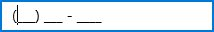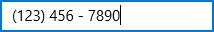## RegEx

Extended Regular expression with more complex expression which gives more usage of input data

We have provided the Optional, Positive and Negative lookahead support in the SfMaskedEdit control.

### Optional:

“*” is the special character that denotes zero or more matches for the preceding character.

Example :

For input “\d\w\d” – The output can be “12” or “1a1” or “123”, because the “\w” is optional. If the user type the input “1a” the character “a” will match with optional mask and the Masked text will be “1a_”. If the mask text is “12” and if the character “3” is typed, then the character “2” will be considered as optional mask and the masked text will be “123”.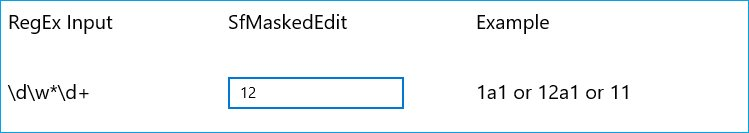Positive Lookahead Regex matches a group after the main expression without including it in the result.

“(?=regex)” is the Positive lookahead regex. It matches only when the regex is matched with the input text.

Example:

For input “(?=123)\d{3}” – It will allow the first two digit and while entering the third digit it will validate the regex and restrict the input. It will allow the output only when the MaskText is “123” for this given input.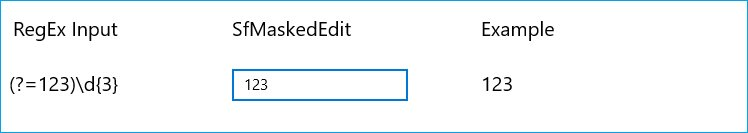Negative Lookahead Regex specifies a group that cannot match after the main expression. If it matches the input text, then the result will be discarded

“(?!regex)” is the Negative lookahead regex. It validates the input on every keypress.

Example:

For input “(?!55)(?!000)(?!666)\d{3}” – \d{3} denotes that the output will be of 3 character length, “(?55)” denotes the value will not begin with “55” and the “(?!000)(?!666)” denotes the output cannot be “000” or “666”.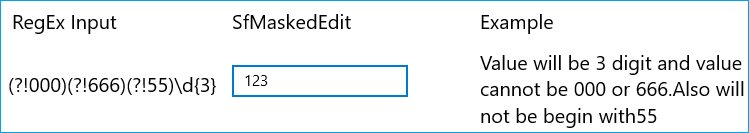Elements Description
abc|def Accepts any one input that matches.
(a|b)+ Accepts the matching input one or more occurrence
[ABC] Accepts any single character included in the specified set of characters.
[^ABC] Accepts any single character not included in the specified set of characters.
[A-Z] Accepts any character between the two specified set of characters inclusive.
(ABC) Matches a group specified within the parenthesis.
\d Accepts any digit. Same as [0-9].
\D Accepts any non digit. Same as [^0-9].
\w Accepts any word character. \w is the same as [a-zA-Z_0-9].
\W Accepts any non word character. \W is the same as [^a-zA-Z_0-9].
\s Accepts any white space characters.
\S Accepts any non white space characters.
\S Accepts any non white space characters.
(?=ABC) Matches a group after the main expression without including it in the result.
(?!ABC) Specifies a group that cannot match after the main expression.
{n} Accepts the input for n number of times.
{n,} Accepts the input for 'n' and more than 'n' number of times.
{n,m} Accepts the input for n minimum number of times and m maximum number of times.
+ Accepts one or more matches for the preceding character.
* Accepts zero or more matches for the preceding character.
? Optional input (Zero or one occurrence of the matching input)
| Acts like a Boolean OR. Matches the expression before or after the |.
. Accepts any character. It can be changed based on culture

For example: The following code example shows the validation for E-mail using Regex Elements.

``<Input:SfMaskedEdit MaskType="RegEx" Mask="[A-Za-z0-9._%-]+@[A-Za-z0-9]+.[A-Za-z]{2,3}" Width="255" Height="46"/>``
``````SfMaskedEdit maskededit = new SfMaskedEdit();